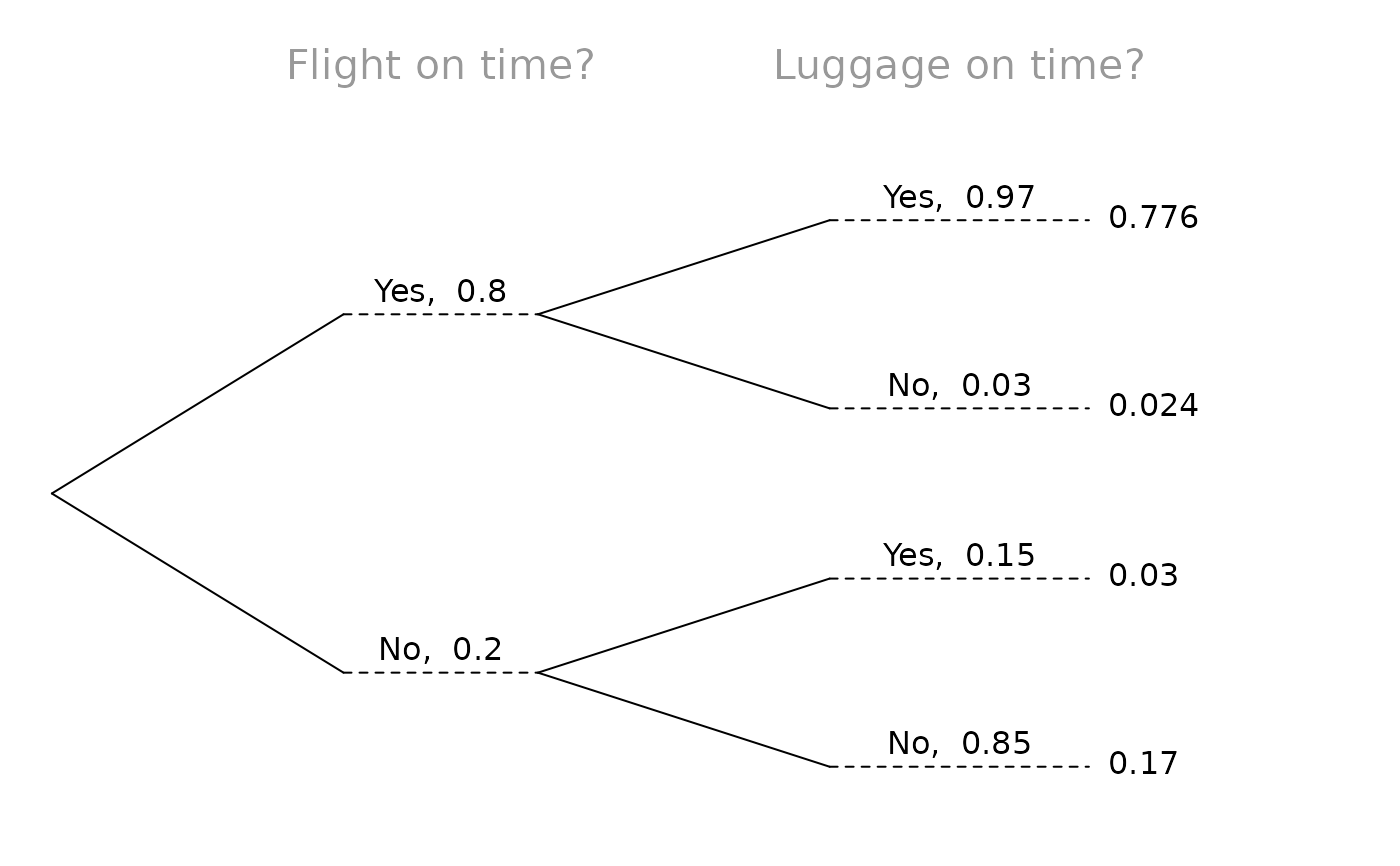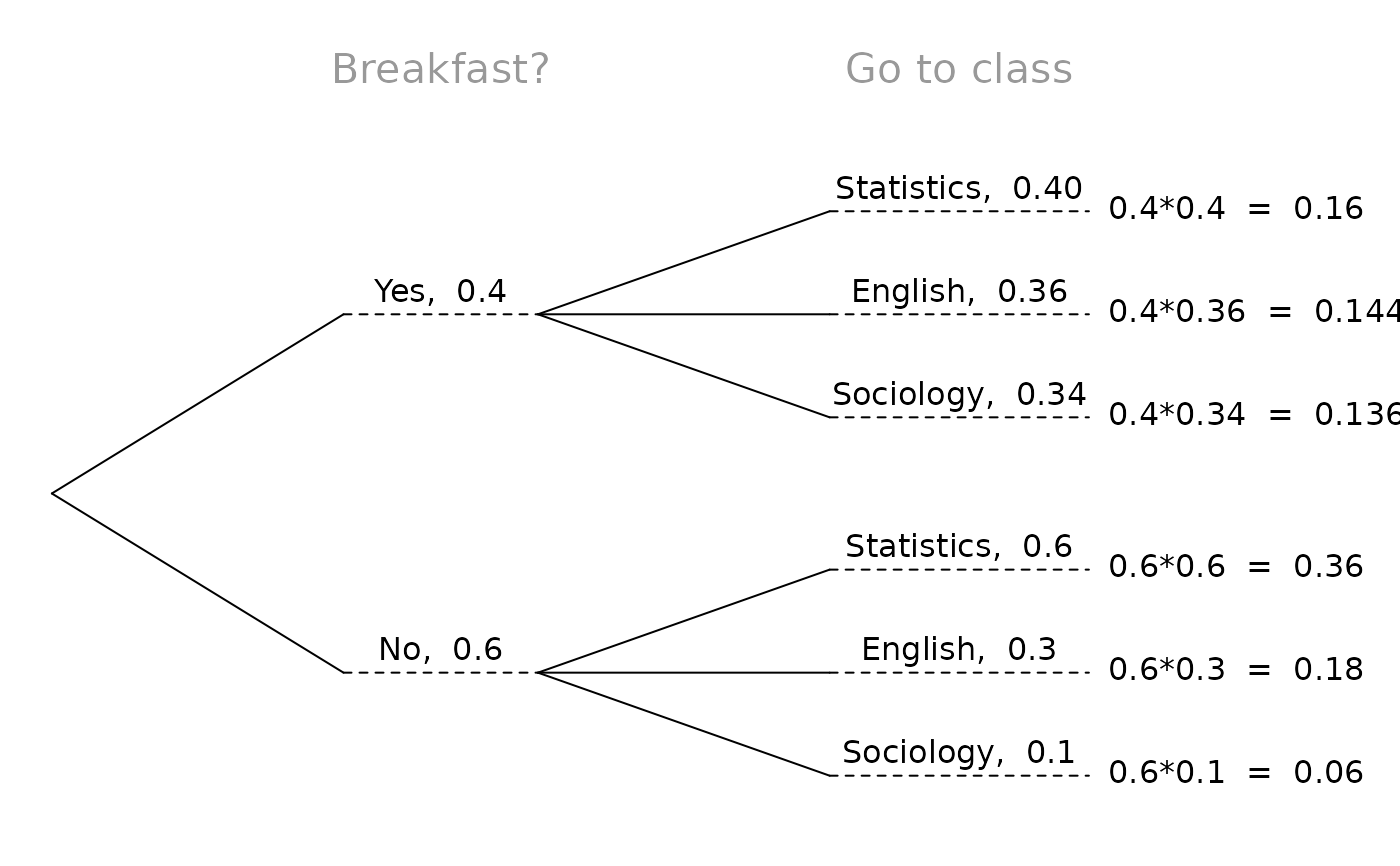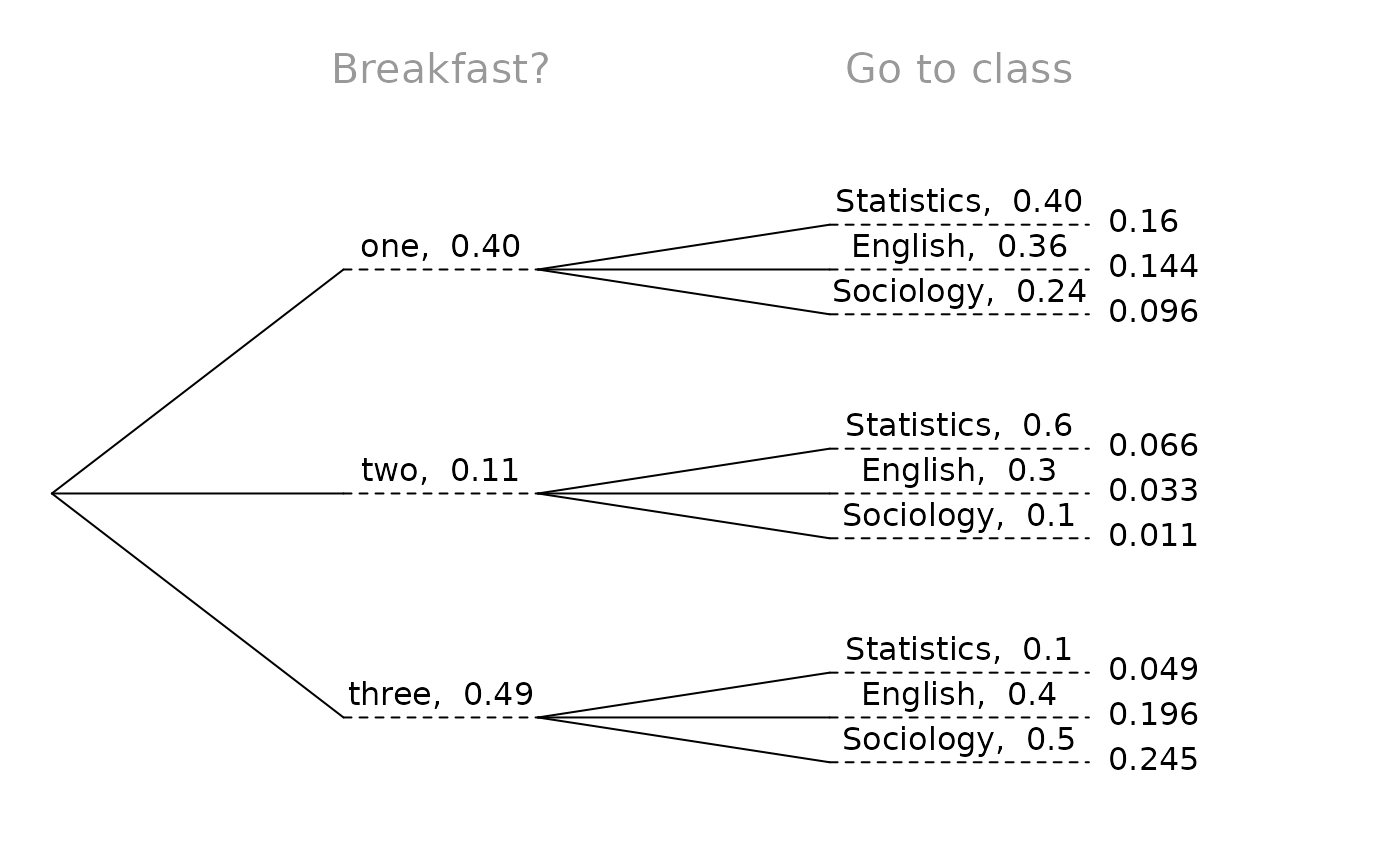Construct beautiful tree diagrams

## Usage

treeDiag(
main,
p1,
p2,
out1 = c("Yes", "No"),
out2 = c("Yes", "No"),
textwd = 0.15,
solwd = 0.2,
SBS = c(TRUE, TRUE),
showSol = TRUE,
solSub = NULL,
digits = 4,
cex.main = 1.3,
col.main = "#999999",
showWork = FALSE
)

## Arguments

main

Character vector with two variable names, descriptions, or questions

p1

Vector of probabilities for the primary branches

p2

List for the secondary branches, where each list item should be a numerical vector of probabilities corresponding to the primary branches of p1

out1

Character vector of the outcomes corresponding to the primary branches

out2

Character vector of the outcomes corresponding to the secondary branches

textwd

The width provided for text with a default of 0.15

solwd

The with provided for the solution with a default of 0.2

SBS

A boolean vector indicating whether to place text and probability side-by-side for the primary and secondary branches

showSol

Boolean indicating whether to show the solution in the tree diagram

solSub

An optional list of vectors corresponding to p2 to list alternative text or solutions

digits

The number of digits to show in the solution

cex.main

Size of main in the plot

col.main

Color of main in the plot

showWork

Whether work should be shown for the solutions

## Author

David Diez, Christopher Barr

## Examples

treeDiag(
c("Flight on time?", "Luggage on time?"),
c(0.8, 0.2), list(c(0.97, 0.03), c(0.15, 0.85))
)treeDiag(c("Breakfast?", "Go to class"), c(.4, .6),
list(c(0.4, 0.36, 0.34), c(0.6, 0.3, 0.1)), c("Yes", "No"),
c("Statistics", "English", "Sociology"),
showWork = TRUE
)treeDiag(
c("Breakfast?", "Go to class"), c(0.4, 0.11, 0.49),
list(c(0.4, 0.36, 0.24), c(0.6, 0.3, 0.1), c(0.1, 0.4, 0.5)),
c("one", "two", "three"), c("Statistics", "English", "Sociology")
)treeDiag(c("Dow Jones rise?", "NASDAQ rise?"),
c(0.53, 0.47), list(c(0.75, 0.25), c(0.72, 0.28)),
solSub = list(c("(a)", "(b)"), c("(c)", "(d)")), solwd = 0.08
)